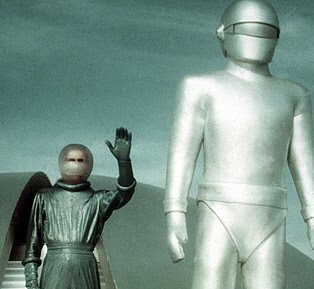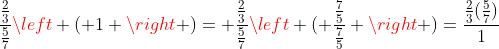## Saturday, 11 September 2010

### Division of Fractions by the Alien Method (and followup)Almost two years ago I wrote about an experience that happened when I let my kids watch an old science fiction movie in class just before Christmas... The blog, and a followup requested by a teacher who admitted he wasn't really sure why the common "divide and multiply method worked... Here they are as a package...
-------------------------------------------------------

The day before Christmas break one of my seminar students brought in the old (1951) video of "The The Day the Earth Stood Still". I worked at my desk as they watched, and about thirty minutes in they called my attention to ask if the math on the blackboard was "real". The Alien in the movie, Klatu(Michael Rennie), in the company of a young boy who lived in the house where he was renting a room, had entered the home of a professor who was supposedly knowledgeable about Astro Physics. I did not recognize any physics I knew from the brief shot of what looked like differential equations of no particular relation, but that could be my limited physics more than the actual images.

I returned to work, but in a few minutes in another scene, Klatu is helping Bobby with his homework and the only line you hear is "All you have to remember is first find the common denominator, and then divide." My head pops up... what were they doing? "Common denominators" leads to thoughts of fractions, but almost no one teaches finding common denominators as a prelude to dividing fractions (which is sort of a shame because it makes division of fractions work like multiplication...the way kids think it should.) It works in fact, if you do not find the common denominator first, but sometimes the answer is as confusing as the problem.

When you multiply fractions, as every fifth grader learns, you just multiply top times top and bottom times bottom... 2/3 x 5/7 = 10/21. The fact that division works the same way is often missed, or misunderstood because it so often leads to nothing simpler... 2/3 divided by 5/7 is indeed (2 divided by 5) over (3 divided by 7) but that seems not to give the classic simple fraction we seek. For some fractions, it will work out fine... if 4/27 is divided by 2/3, the answer is (four divided by two ) over (27 divided by 3) = 2/9 and that is the answer you get by the method you memorized (but never understood, most likely) in the fifth grade.

But what if we follow the advice of the alien Klatu. If we convert 2/3 and 5/7 to fractions with a common denominator, we get 14/21 and 15/21, and if we divide top by top and bottom by bottom we get 14/15) over 1, which is just 14/15... job done...

I can imagine including some visuals and suggestive images to help it make sense... It is after all, just a reversal of the multiplication process. If we say "3 dogs times 5 = 15 dogs" then by division we should have the equivalent expressions that "15 dogs divided by 5 = 3 dogs." and just as naturally "15 dogs divided by 3dogs = 5" . Students who have learned (I've been in England too long, I just had to edit "learnt") that "eighths" and "fifths" are just units like "dogs" and "kittens" should then understand that 5 eighths divided by three eighths is just as clearly 5/3.

A few days after I wrote that blog I got a response that asked, more or less, "OK, why does the common algorithm work?"
This was my response

I want to make one comment about division of fractions that seems harder to visulaize than for general division, and then I hope to explain in simple terms just why "invert and multiply" works.

For every multiplication problem, there are two associated division problems; A x B = C begets C/A=B and C/B=A. Elementary teachers call these a "family of facts for C" (or did in the recent past.. educational language changes too fast for firm statments by a non-elementary teacher). So if we add units to one or both factors, appropriate units must be appended to the product. So how does this effect operations with fractions? Well if we have length, as in ANON's comment, then the division problem he states, "If we think of it as a piece of wood with length 2/3, then I believe the question is how many 5/7's are there in the piece of wood" he is dividing length by length to get a pure scaler counting how many pieces (or fractions of a piece) will fit into another. In the case he gives, the answer would be only 14/15 of a piece... becuase the 2/3 unit length is not quite enough to provide a 5/7 unit length piece...

The multiplication associated with this operation is then 14/15 of 5/7 units = 2/3 units... What about the other division in this family of facts... 2/3 units divided by 14/15 (a scaler here, not a length)will give 5/7 units length. What is this sitution describing? This seems the one most difficult for teachers and students alike. We all know what it means to divide a length into (by?) two pieces, but what sense does it make to divide it into 1/2 a piece.

We might try to make this clear to students by taking some common length (12 inches?) and see what happens if we divide it into (by) 8 pieces, then four, then two, then one, (each division is by half the previousl number)and look at the pattern of lengths. 12/8=3/2; 12/4 = 3; 12/2 = 6; 12/1= 12... I am confident most students could identify the next numbers in the sequence, 12/ (1/2) = 24, and 12/(1/4) = 48.

At this point, using whole numbers as divisors, the pattern for "invert and multiply" seems obvious, but this is far from a why for all fraction problems.

Let's look at one more case where we sneak in a related idea at the elementary level. Given a problem like 3.5 divided by .04, the student is taught to "move the decimal places enough to make the divisor (.04) a whole number. What we do is another problem (350 divided by 4) that has the same answer (87.5)as the original. Another why does that work that is not often explained.

What do the two operations have in common.... multiplication by one. In each case we have a division (fraction) operation and we simply mulitiply the fraction by a carefully chosen version of one that will make it easier to do. If we view 3.5/.04 as a fraction, then every fifth grader knows that multipliying it by one will not change its value. This is the core of what we do to find equivalent fractions... to get 3/5 = 6/10 we multiply by one, but expressed as 2/2... The decimal division problem uses the same approach... we multiply 3.5/.04 by 100/100 to get another name for the same fraction, 350/4.

Now to explain "invert and multiply" we just use the same idea... dividing fractions is simply fractions which have fractions instead of integers in the numerator and denominator. We want to multiply by one in a way that the division problem will be easier. But the easiest number to divide by is one,... so why not pick a number that changes the denominator of the fraction over a fraction to be a one... that is, multiply by its reciprocal. So for 2/3 divided by 5/7 we can write(Pardon the duplication, I'm trying a new latex editor to replace some images...
)

And I hope that makes it clear....Anonymous said...

Another way to explain:

2/3 div by 5/7 means how many sets of 5/7 are there in 2/3.

First find out how many 1/7 there are, then group those in sets of 5:

How many sevenths in any number? 7 times as many as the number, so...

2/3 div by 1/7 = 2/3 times 7

Now, to find out how many sets of 5 sevenths, we divide by 5, so...

(2/3 x 7) div by 5 = 2/3 x 7/5
which shows that
2/3 div by 5/7 = 2/3 x 7/5

There's nothing special about the numbers in the problem, so this method will work for any numbers.

Unknown said...

It is of course also possible to interpret the division of decimals as making them first to have common denominators.

3.5/0.04 = (35/10) / (4/100) = (350/100) / (4/100) = 350/4.

Unknown said...

I always thought of it by the definition of the reciprocal. A reciprocal is how many of a certain fraction fit into one whole, and division is always asking "how many of these fit inside of that?"

A question like 2/3 div by 5/7 is really "how many 5/7 fit into 2/3?"

Knowing that 5/7 fits into 1 7/5 of a time, then 5/7 fits into 2/3 7/5 of 2/3 times, hence the flip and the multiplication.